# Analytic Wavelet Transform (Advanced Signal Processing Toolkit)

LabVIEW 2014 Advanced Signal Processing Toolkit Help

Edition Date: June 2014

Part Number: 372656C-01

»View Product InfoDownload Help (Windows Only)

The analytic wavelet transform (AWT) is a wavelet transform that provides both the magnitude and phase information of signals in the time-scale or time-frequency domain. The magnitude information returned by the AWT describes the envelopes of signals. The phase information encodes the time-related characteristics of signals, for example, the location of a cusp. You usually use the magnitude information for time-frequency analysis and phase information for applications such as instantaneous frequency estimation.

The AWT computes the inner products of the analyzed signal and a set of complex Morlet wavelets. This transform is called the analytic wavelet transform because the complex Morlet wavelets are analytic, that is, the power spectra of the Morlet wavelets are zero at negative frequencies. The resulting AWT coefficients are complex numbers. These coefficients measure the similarity between the analyzed signal and the complex Morlet wavelets. The AWT is just one type of complex continuous wavelet transform.

Use the WA Analytic Wavelet Transform VI to compute the AWT.

## Scale and Frequency

Wavelets are functions of time and scale, so you can consider a wavelet transform as a tool that produces a time-scale representation of signals. You also can consider the time-scale representation of signals as a time-frequency representation, because wavelets with different scales measure the corresponding frequency components in the signal. The frequency of a wavelet is inversely proportional to the scale factor.

Using the WA Analytic Wavelet Transform VI, you can specify different settings for the scale factor to compute the AWT. When you set scale sampling method to even scale, this VI computes the wavelet coefficients at evenly-distributed integer scales. You usually use the even scale option to obtain the time-scale representation of a signal. When you set scale sampling method to even frequency, this VI computes the wavelet coefficients at scales with evenly-distributed frequencies. Notice that the scales are not evenly-distributed. You usually use the even frequency option to obtain the time-frequency representation of a signal.

Because the time and frequency resolutions of wavelets are adaptive, the AWT provides adaptive time and frequency resolutions. Conventional time-frequency analysis methods, such as the short-time Fourier transform (STFT), only provide uniform time and frequency resolutions in the whole time-frequency domain.

The following figure shows a common wavelet test signal, the HypChirps signal. This signal contains two frequency components, which are hyperbolic functions over time. The frequency components change slowly at the beginning and rapidly at the end.The following figure shows two representations of this HypChirps signal in the time-frequency domain based on the STFT method.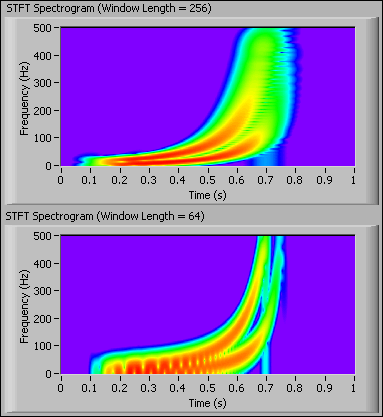In the previous figure, if you use a relatively long window, 256, you obtain fine frequency resolution and coarse time resolution. Therefore, you can distinguish the frequency components of the HypChirps signal at lower frequencies with a long window. If you use a short window, 64, you obtain coarse frequency resolution and fine time resolution. Therefore, you can distinguish the frequency components of the HypChirps signal at higher frequencies with a short window. However, you cannot distinguish the two frequency components at both low and high frequencies in either of the STFT spectrograms.

The following figure shows the tiling of the STFT-based time-frequency representation.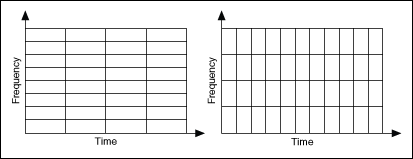In the previous figure, you can see that the STFT spectrogram has uniform time-frequency resolution across the whole time-frequency domain. You can balance the time-frequency resolution by adjusting the window length. The left tiling diagram provides better frequency resolution in the STFT Spectrogram (Window Length = 256) graph of the STFT spectrograms of the HypChirps signal. The right tiling diagram shows better time resolution in the STFT Spectrogram (Window Length = 64) graph of STFT spectrograms of the HypChirps signal. However, you cannot achieve high time resolution and frequency resolution simultaneously.

The following figure shows the AWT-based time-frequency representation of the HypChirps signal. In the Scalogram graph, you can distinguish the two frequency components at both low and high frequencies.The following figure shows the tiling of the AWT-based time-frequency representation that provides fine frequency resolution at low frequencies and fine time resolution at high frequencies.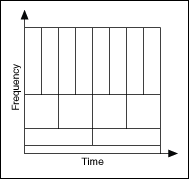Similar to the continuous wavelet transform (CWT), the AWT also adds excess information redundancy and is computationally intensive. Moreover, you cannot reconstruct the original signal from the AWT coefficients.

## Wavelet Normalization: Energy versus Amplitude

In wavelet analysis, wavelets at different scales often have the same energy. Because both the center frequency and the bandwidth of a wavelet are inversely proportional to the scale factor, the wavelet at a larger scale has a higher magnitude response than a wavelet at a smaller scale. The following figure shows the Fourier magnitude spectra of different wavelets with energy normalization.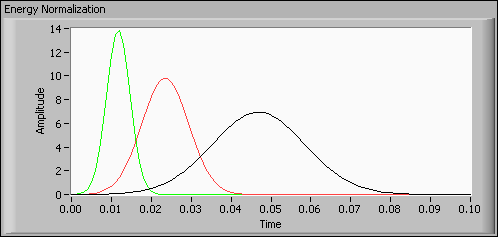However, some real-world applications require that you use a uniform amplitude response to measure the exact amplitude of the signal components, as shown in the following figure.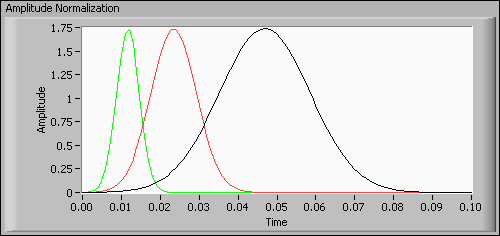With the WA Analytic Wavelet Transform VI, you can analyze a signal based on amplitude normalization by selecting amplitude in the normalization list. If you set scale sampling method to even frequency and set normalization to amplitude, the WA Analytic Wavelet Transform VI generates the scalogram of the HypChirps signal, as shown in the following figure.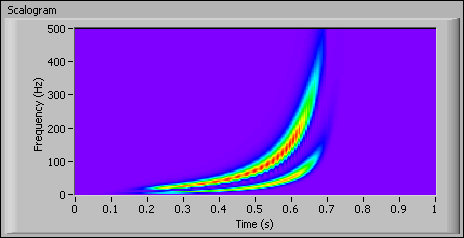Notice that the magnitude at high frequencies (small scales) also has been enlarged. With amplitude normalization, you can obtain the precise magnitude evolution over time for each hyperbolic chirp.